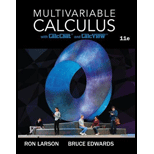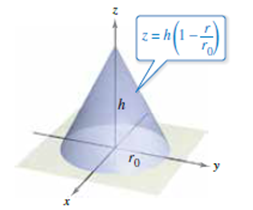# Using Cylindrical Coordinates In Exercises 23-28, use cylindrical coordinates to find the indicated characteristic of the cone shown in the figure. Find the center of mass of the cone, assuming that its density at any point is proportional to the distance between the point and the base. Use a computer algebra system to evaluate the triple integral.### Multivariable Calculus

11th Edition
Ron Larson + 1 other
Publisher: Cengage Learning
ISBN: 9781337275378

#### Solutions

Chapter
Section### Multivariable Calculus

11th Edition
Ron Larson + 1 other
Publisher: Cengage Learning
ISBN: 9781337275378
Chapter 14.7, Problem 26E
Textbook Problem
1 views

## Using Cylindrical Coordinates In Exercises 23-28, use cylindrical coordinates to find the indicated characteristic of the cone shown in the figure.Find the center of mass of the cone, assuming that its density at any point is proportional to the distance between the point and the base. Use a computer algebra system to evaluate the triple integral.

This textbook solution is under construction.

### Still sussing out bartleby?

Check out a sample textbook solution.

See a sample solution

#### The Solution to Your Study Problems

Bartleby provides explanations to thousands of textbook problems written by our experts, many with advanced degrees!

Get Started